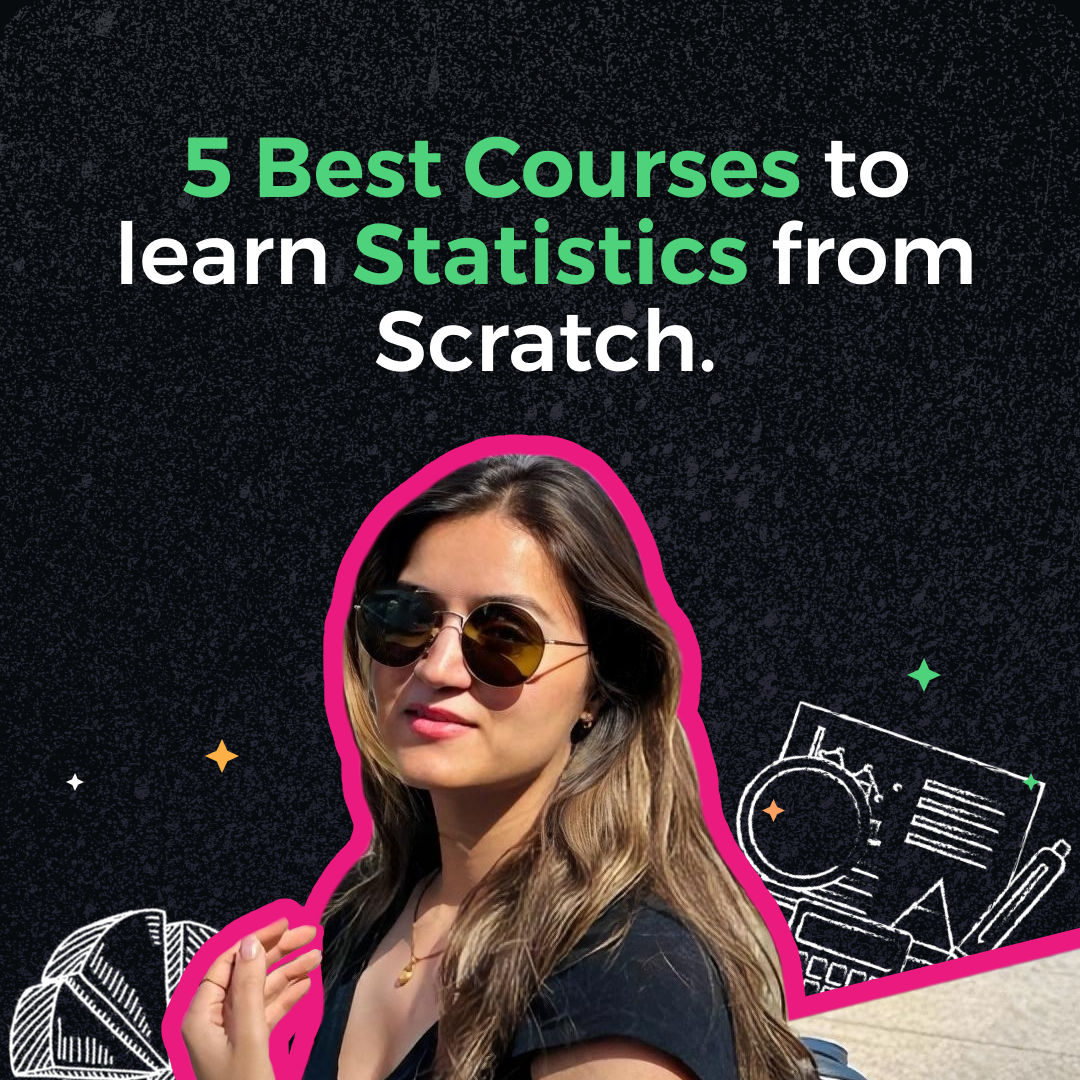# Best courses to learn Statistics for Data Science in 2023: For beginnersStatistics is an integral part of data science and plays a crucial role in making sense of the vast amount of data generated in today's world. Whether you're a beginner looking to build a solid foundation or an intermediate learner seeking to enhance your statistical skills, Let's explore four remarkable courses that cater to learners at different levels and durations, offering invaluable insights into statistical concepts and their real-world applications.

## 1. Introduction to statistics offered by Stanford on Coursera

Level: Beginner

Duration: Approx 14 hours

Fee: Free to audit

What you’ll learn: Exploratory Data Analysis, Descriptive Statistics, Sampling and Randomized Controlled Experiments Sampling, Probability, Sampling Distributions and the Central Limit Theorem, Regression, Common Tests of Significance, Resampling, Multiple Comparisons

About the course: In Stanford's "Introduction to Statistics," you'll learn how to think critically about data and make sense of it. By the end of the course, you'll be able to explore data, understand how to gather it properly, and use tests to find important patterns. These essential skills will set you up for further studies in statistical thinking and machine learning.

## 2. Data Analysis with R Specialization by Duke University on Coursera

Level: Beginner

Duration: Approx 5-6 hours

Fee: Free to audit

What you’ll learn: Statistics, Linear Regression, R Programming, Regression Analysis, Rstudio, Exploratory Data Analysis, Statistical Inference, Statistical Hypothesis Testing

About the course: In this Specialization, you'll master analyzing and visualizing data using R. You'll create reports that others can reproduce, and learn about statistical inference to make data-driven decisions. Effectively communicate your findings without using complex statistical terms. You'll also be able to evaluate claims based on data and work with R packages to manipulate and present data visually.

## 3. Harvard University: Statistics and R on edx

Level: Intermediate

Duration: 4 weeks (2-4 hours/week)

Fee: Free to audit

What you’ll learn: Random variables, Distributions, Inference: p-values and confidence intervals, Exploratory Data Analysis, Non-parametric statistics

About the course: In this course, you'll learn the R programming language specifically for statistical data and analysis in the life sciences.

You'll grasp the basics of statistical inference, understanding how to compute p-values and confidence intervals while using R code to analyze data.

Course also has ample clear examples that bridge the gap between concepts and practical implementation. To test your understanding and data analysis skills, you'll have problem sets with R programming exercises.

Through visualization techniques, you'll explore new data sets and choose the best approach for analysis. We'll also introduce robust statistical methods as alternatives when data deviate from standard assumptions. By utilizing R scripts for data analysis, you'll gain a solid foundation in reproducible research practices.

## 4. Statistics with Python Specialization by University of Michigan on Coursera

Level: Beginner

Duration: 3 months (5 hours/week)

Fee: Free to audit

What you’ll learn: Python Programming, Data Visualization (DataViz), Statistical Model, Statistical inference methods

About the course: This Specialization consists of 3 courses namely:

This specialization aims to teach you the basics and intermediate concepts of statistical analysis using Python. You'll discover where data comes from, learn about different data types, and explore data design and management. Effectively exploring and visualizing data will be a key skill you'll develop.

You'll also master using data for estimation and testing theories, creating confidence intervals, interpreting inferential results, and applying advanced statistical modeling techniques. By the end, you'll understand how to link research questions with the statistical and data analysis methods you've learned, making your analyses more insightful and valuable.